# scipy.signal.find_peaks¶

scipy.signal.find_peaks(x, height=None, threshold=None, distance=None, prominence=None, width=None, wlen=None, rel_height=0.5)[source]

Find peaks inside a signal based on peak properties.

This function takes a one-dimensional array and finds all local maxima by simple comparison of neighbouring values. Optionally, a subset of these peaks can be selected by specifying conditions for a peak’s properties.

Parameters: x : sequence A signal with peaks. height : number or ndarray or sequence, optional Required height of peaks. Either a number, None, an array matching x or a 2-element sequence of the former. The first element is always interpreted as the minimal and the second, if supplied, as the maximal required height. threshold : number or ndarray or sequence, optional Required threshold of peaks, the vertical distance to its neighbouring samples. Either a number, None, an array matching x or a 2-element sequence of the former. The first element is always interpreted as the minimal and the second, if supplied, as the maximal required threshold. distance : number, optional Required minimal horizontal distance (>= 1) in samples between neighbouring peaks. The removal order is explained in the notes section. prominence : number or ndarray or sequence, optional Required prominence of peaks. Either a number, None, an array matching x or a 2-element sequence of the former. The first element is always interpreted as the minimal and the second, if supplied, as the maximal required prominence. width : number or ndarray or sequence, optional Required width of peaks in samples. Either a number, None, an array matching x or a 2-element sequence of the former. The first element is always interpreted as the minimal and the second, if supplied, as the maximal required prominence. wlen : number, optional Used for calculation of the peaks prominences, thus it is only used if one of the arguments prominence or width is given. See argument wlen in peak_prominences for a full description of its effects. rel_height : float, optional Used for calculation of the peaks width, thus it is only used if width is given. See argument rel_height in peak_widths for a full description of its effects. peaks : ndarray Indices of peaks in x that satisfy all given conditions. properties : dict A dictionary containing properties of the returned peaks which were calculated as intermediate results during evaluation of the specified conditions: ‘peak_heights’ If height is given, the height of each peak in x. ‘left_thresholds’, ‘right_thresholds’ If threshold is given, these keys contain a peaks vertical distance to its neighbouring samples. ‘peak_prominences’, ‘right_bases’, ‘left_bases’ If prominence is given, these keys are accessible. See peak_prominences for a description of their content. ‘width_heights’, ‘left_ips’, ‘right_ips’ If width is given, these keys are accessible. See peak_widths for a description of their content. To calculate and return properties without excluding peaks, provide the open interval (None, None) as a value to the appropriate argument (excluding distance).

find_peaks_cwt
Find peaks using the wavelet transformation.
peak_prominences
Directly calculate the prominence of peaks.
peak_widths
Directly calculate the width of peaks.

Notes

In the context of this function, a peak or local maximum is defined as any sample whose two direct neighbours have a smaller amplitude. For flat peaks (more than one sample of equal amplitude wide) the index of the middle sample is returned (rounded down in case the number of samples is even). For noisy signals the peak locations can be off because the noise might change the position of local maxima. In those cases consider smoothing the signal before searching for peaks or use other peak finding and fitting methods (like find_peaks_cwt).

• Almost all conditions (excluding distance) can be given as half-open or closed intervals, e.g 1 or (1, None) defines the half-open interval $$[1, \infty]$$ while (None, 1) defines the interval $$[-\infty, 1]$$. The open interval (None, None) can be specified as well, which returns the matching properties without exclusion of peaks.
• The border is always included in the interval used to select valid peaks.
• For several conditions the interval borders can be specified with arrays matching x in shape which enables dynamic constrains based on the sample position.
• The order of arguments given in the function definition above mirrors the actual order in which conditions are evaluated. In most cases this order is the fastest one because faster operations are applied first to reduce the number of peaks that need to be evaluated later.
• Satisfying the distance condition is accomplished by iterating over all peaks in descending order based on their height and removing all lower peaks that are too close.
• Use wlen to reduce the time it takes to evaluate the conditions for prominence or width if x is large or has many local maxima (see peak_prominences).

Warning

This function may return unexpected results for data containing NaNs. To avoid this, NaNs should either be removed or replaced.

New in version 1.1.0.

Examples

To demonstrate this function’s usage we use a signal x supplied with SciPy (see scipy.misc.electrocardiogram). Let’s find all peaks (local maxima) in x whose amplitude lies above 0.

>>> import matplotlib.pyplot as plt
>>> from scipy.misc import electrocardiogram
>>> from scipy.signal import find_peaks
>>> x = electrocardiogram()[2000:4000]
>>> peaks, _ = find_peaks(x, height=0)
>>> plt.plot(x)
>>> plt.plot(peaks, x[peaks], "x")
>>> plt.plot(np.zeros_like(x), "--", color="gray")
>>> plt.show()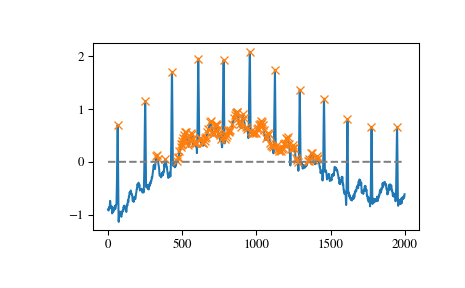We can select peaks below 0 with height=(None, 0) or use arrays matching x in size to reflect a changing condition for different parts of the signal.

>>> border = np.sin(np.linspace(0, 3 * np.pi, x.size))
>>> peaks, _ = find_peaks(x, height=(-border, border))
>>> plt.plot(x)
>>> plt.plot(-border, "--", color="gray")
>>> plt.plot(border, ":", color="gray")
>>> plt.plot(peaks, x[peaks], "x")
>>> plt.show()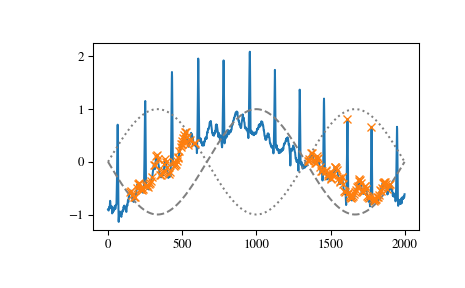Another useful condition for periodic signals can be given with the distance argument. In this case we can easily select the positions of QRS complexes within the electrocardiogram (ECG) by demanding a distance of at least 150 samples.

>>> peaks, _ = find_peaks(x, distance=150)
>>> np.diff(peaks)
array([186, 180, 177, 171, 177, 169, 167, 164, 158, 162, 172])
>>> plt.plot(x)
>>> plt.plot(peaks, x[peaks], "x")
>>> plt.show()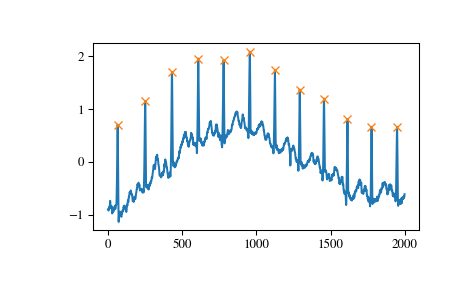Especially for noisy signals peaks can be easily grouped by their prominence (see peak_prominences). E.g. we can select all peaks except for the mentioned QRS complexes by limiting the allowed prominenence to 0.6.

>>> peaks, properties = find_peaks(x, prominence=(None, 0.6))
>>> properties["prominences"].max()
0.5049999999999999
>>> plt.plot(x)
>>> plt.plot(peaks, x[peaks], "x")
>>> plt.show()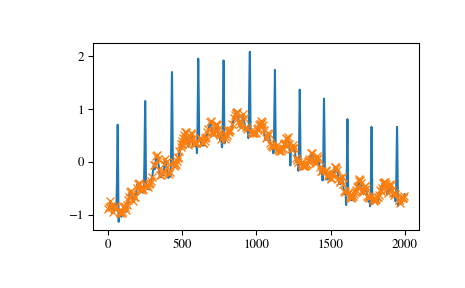And finally let’s examine a different section of the ECG which contains beat forms of different shape. To select only the atypical heart beats we combine two conditions: a minimal prominence of 1 and width of at least 20 samples.

>>> x = electrocardiogram()[17000:18000]
>>> peaks, properties = find_peaks(x, prominence=1, width=20)
>>> properties["prominences"], properties["widths"]
(array([1.495, 2.3  ]), array([36.93773946, 39.32723577]))
>>> plt.plot(x)
>>> plt.plot(peaks, x[peaks], "x")
>>> plt.vlines(x=peaks, ymin=x[peaks] - properties["prominences"],
...            ymax = x[peaks], color = "C1")
>>> plt.hlines(y=properties["width_heights"], xmin=properties["left_ips"],
...            xmax=properties["right_ips"], color = "C1")
>>> plt.show()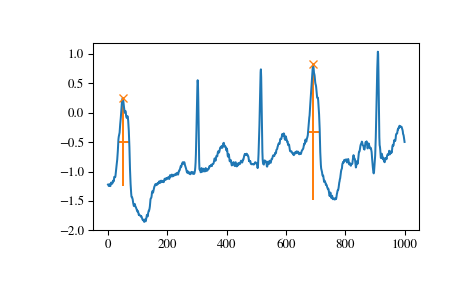#### Previous topic

scipy.signal.argrelextrema

#### Next topic

scipy.signal.find_peaks_cwt